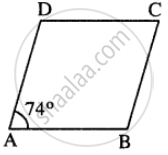# In Rhombus Abcd; (I) If ∠A = 74° ; Find ∠B and ∠C. (Ii) If Ad = 7.5 Cm ; Find Bc and Cd. - Mathematics

Sum

In rhombus ABCD;
(i) if ∠A = 74° ; find ∠B and ∠C.
(ii) if AD = 7.5 cm ; find BC and CD.

#### Solution

∠A + ∠B = 180°
74° + ∠B = 180°
∠B =180° – 74°= 106°opposite angles of Rhombus are equal.

∠A = ∠C = 74°

Sides of Rhombus are equal.

BC = CD = AD = 7.5 cm

(i) ∠B = 106° ; ∠C = 74°

(ii) BC = 7.5 cm and CD = 7.5 cm

Is there an error in this question or solution?
Chapter 17: Special Types of Quadrilaterals - Exercise 17 [Page 198]

#### APPEARS IN

Selina Concise Mathematics Class 8 ICSE
Chapter 17 Special Types of Quadrilaterals
Exercise 17 | Q 3 | Page 198
Share